×#### Thank you for registering.

One of our academic counsellors will contact you within 1 working day.

Click to Chat

1800-1023-196

+91-120-4616500

CART 0

• 0

MY CART (5)

Use Coupon: CART20 and get 20% off on all online Study Material

ITEM
DETAILS
MRP
DISCOUNT
FINAL PRICE
Total Price: Rs.

There are no items in this cart.
Continue ShoppingArea as Definite Integral

Table of Content

Introduction to Area as Definite Integral

Geometrical Interpretation of definite integral

Working Rules for finding the Area

Related Resources

Introduction to Area as Definite Integral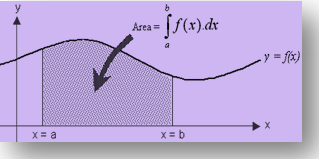One of the major applications of definite integral is finding the area enclosed by curves. Definite integrals are used for finding the accumulated area. It can be used to compute the area enclosed under, between or below the curves. In case of a strictly positive function, the area enclosed by it with the x-axis is given by simply the definite integral.Similarly, if the function is negative, the area is (-1) times the definite integral. Moreover, if we wish to find the area between two positive functions, then the required area is given by (higher function – lower function). Both these cases have been illustrated in the figure given below:

Let us assume the function f(x) to be continuous in [a, b]. Then the area bounded by the curve y = f(x), x-axis and the lines x = a and x = b is given by the formula

provided f (x) > 0 (or f(x) < 0) ∀="" x ∈="" (a="" ,="">

Note:

At times, it becomes convenient to regard x as a function of y and hence use the formula for area with respect to y.

The area enclosed between x = f(y), y axis and the lines y = c and y = d is given byIf we have two functions f(x) and g(x) such that f(x) < g(x) ∀="" x ∈ [a,="" b],="" then="" the="" area="" bounded="" by="" the="" curves="" y="f(x)," y="g(x)" and="" lines="" x="a, x" =="" b="" (a="">< b)="" is="" given="">

?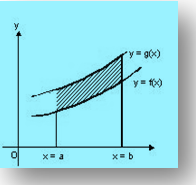Various other possible cases have been discussed in the section area under curves. We now throw some light on the geometrical interpretation of Definite Integral:

Geometrical Interpretation of definite integral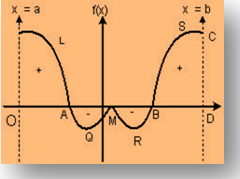If f(x) > 0 for all x ∈ [a, b]; then  is numerically equal to the area bounded by the curve y = f(x), the x-axis and the straight lines x = a and x = b i.e.

In general  represents the algebraic sum of the figures bounded by the curve y = f(x), the x-axis and the straight line x = a and x = b. The areas above x-axis are taken with a positive sign and the areas below x-axis are taken with a negative sign i.e.,

area OLA – area AQM – area MRB + area BSCD

Note:

represents algebraic sum of areas which means that if area of function y = f(x) is asked between a to b, then

⇒ Area bounded =   and is not represented by .

Eg: If someone asks the area of y = x3 between -1 to 1.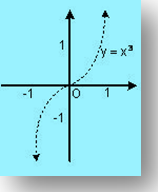Then y = x3 could be plotted as given in the figure above. In that case, we have the area as:

Area =

We can also derive the area using the above definition

Area =

=

= ½.

Hence, students are advised to note that the answer obtained in both the cases is same.Now, we see what happens when we integrate the function from -1 to 1, does it also yield the same result?

We integrate the function f(x) = x3 from -1 to 1:

A =

Hence, this does not represent any area. This clearly shows the difference between the definite integral and the area.

Students are advised to carefully note the difference between the definite integral and the area as majority of the students often confuse these concepts with each other.

Working Rules for finding the Area

If a curve lies completely above the x axis, then the area is positive but when it lies completely below x axis, then the area is negative. However we have the convention to consider the magnitude only.In the figure given above, the curve lies on both sides of x-axis. In such a case, the area is obtained by calculating both the areas separately and then adding their moduli.

Hence, if the curve y = f(x) crosses the x -axis n times when x varies from a to b, then the area between y = f(x), x-axis and the lines x = a and x = b is given by

A = |A1| + |A2| + …...... + |An|.

If the curve is symmetrical about x axis, or y axis, or both, then calculate the area of one symmetrical part and multiply it by the number of symmetrical parts to get the whole area.

The various other working rules of finding area have been discussed in detail in the section “area under curves”.

We now discuss some of the illustrations based on these concepts:

Illustration 1:

Find the area between the curves y = x2 + x – 2 and y = 2x, for which |x2+ x – 2| + | 2x | = |x2 + 3x – 2| is satisfied.

Solution:

The given curves are y = x2 + x - 2 and y = 2x

Now, |x2 + x - 2| + |2x| = |x2 + 3x -2| ⇒ (x2 + x - 2) and 2x have same sign

Thus required area is

ar (PQR) + ar (SNT)Solving this we get the required area as

= 7/6 + [10/3 –13/6] = 7/6 + 7/6 = 7/3.

_____________________________________________________________

Illustration 2:

Find out the area enclosed by circle |z| = 2, parabola y = x2 + x + 1, the curve y = [sin2 x/4 + cos x/4] and x-axis (when [.] is the greatest integer function).

Solution:

For x ∈ [-2, 2]

⇒ 1 <>2 x/4 + cos x/4 <>∴ [sin2 x/4 + cos x/4] = 1

Now we have to find out the area enclosed by the circle |z| = 2, parabola (y - 3/4) = (x+1/2)2, line y = 1 and the x-axis.

∴ Required area is the shaded area in the figure.

Hence required area =

Solving this yields the required area as

= (2π/3 + √3 – 1/6) sq. units.

_________________________________________________________________

Illustration 3:

Let f(x) = Max. {sin x, cos x, 1/2} then determine the area of the region bounded by the curves y = f(x), x-axis, y-axis and x = 2π.

Solution: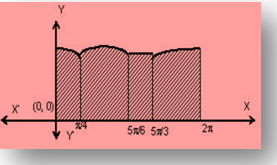f(x) = Max {sin x, cos x, 1/2}

interval value of f(x) for 0 < x>< π is="" cos="">

for  π/4 < x>< 5π/6="" the="" value="" is="" sin="">

for 5π/6 < x>< 5π/3,="" the="" value="" is="">

for 5π/3 <>< 2π cos="">

Hence required area is

= (5π/12 + √2 + √3) sq units.

________________________________________________________________________

Illustration 4:

Find area bounded by y = |4 – x2|/4 and y = 7 - |x|.

Solution:y = |4 – x2|/ 4 ….. (1)

y = 7 – |x|      …....(2)

Rewriting (I) and (II)

y = {(4 – x2)/4, – 2<><>

= (x2 – 4)/4, x ∈ (–∞, –2) U (2, ∞)

y = {7 – x, x > 0

7 + x,  x <>

Required area (PQRSTUP) = 2 area (PQRSP)

= 2 [area of (OVRSO) – area of (POQP) – area of (QRVQ)]

= 2 [1/2 (7 + 3)4 - ∫20 (4 – x2)/4) dx – ∫42 (x2–4)/4 dx]

= 2 [18 + 2/3 – 16/3 + 4 + 2/3 – 2]

= 32 sq unit.

_______________________________________________________________

Illustration 5:

Let f be a real valued function satisfying f(x/y) and limx→0 f(1 + x)/x = 3. Find the area bounded by the curve y = f(x), the y axis and the line y = 3

Solution:

Given = f(x/y) = f(x) – f(y)                                                                        .... (1)

Putting x = y = 1, we get f(1) = 0

Now, f’(x) = lim h→0 [f(x + h) – f(x)] / h

= lim h→0 f(1 + h/x) /h (from(1))

= lim h→0 f(1 + h/x) (h/x)x

⇒ f'(x) = 3/x  {since limx→0 f(1+x)/x = 3}

⇒ f(x) = 3 ln x + c

Putting x = 1

⇒ c = 0

⇒ f(x) = 3 ln x = y (say)

Required area = ∫3–∞ x dy = ∫3–∞ ey/3 dy

This yields the required area as

= 3 (e – 0) = 3e sq. units.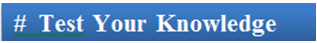Q1. If the function is negative, then area is:

(a) (-1) times the definite integral

(b) same as the definite integral

(c) may or may not be the same as definite integral

(d) there is no relation between the area and the definite integral.

Q2.  is numerically equal to the area

(a) bounded by the curve x = f(y) from a to b.

(b) bounded by the curve y = f(x) from b to a.

(c) bounded by the curve y = f(x), the y-axis and the straight lines x = a and x = b.

(d) bounded by the curve y = f(x), the x-axis and the straight lines x = a and x = b.

Q3. If we have two functions f(x) and g(x) such that f(x) < g(x) ∀="" x ∈ [a,="" b],="" then="" the="" area="" bounded="" by="" the="" curves="" y="f(x)," y="g(x)" and="" lines="" x="a, x" =="" b="" (a="">< b)="" is="" given="">

(a) ?

(b)

(c)

(d)

Q4. If the curve is symmetrical about x axis, or y axis, or both, then calculate

(a) the area of all symmetrical parts and multiply it by 2 to get the whole area.

(b) the area of one symmetrical part and multiply it by the number of symmetrical parts to get the whole area.

(c) the area of one symmetrical part and divide it by the number of symmetrical parts to get the whole area.

(d) calculate the area as you would have done evven if there would not have any symmetrical part.

Q5. Area of function y = f(x) is asked between a to b, then

(a) Area bounded =   and is not represented by .

(b) Area bounded is not represented by   and is given by .

(c) Area bounded =   and is not represented by .

(d) Area bounded is simply  and there is no need of modulus.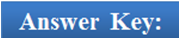Q1
Q2
Q3
Q4
Q5

(a)
(d)
(c)
(b)
(a)

Related Resources

You may wish to refer indefinite integral.

For getting an idea of the type of questions asked, refer the previous year papers.

To read more, Buy study materials of Definite integral comprising study notes, revision notes, video lectures, previous year solved questions etc. Also browse for more study materials on Mathematics here.### Course Features

• 731 Video Lectures
• Revision Notes
• Previous Year Papers
• Mind Map
• Study Planner
• NCERT Solutions
• Discussion Forum
• Test paper with Video Solution